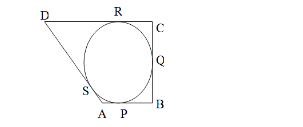# A quadrilateral ABCD is drawn to circumscribe a circle.`
Question:

A quadrilateral ABCD is drawn to circumscribe a circle. Prove that sums of opposite sides are equal.

Solution:We know that the tangents drawn from an external point to a circle are equal.

$\therefore A P=A S \ldots \ldots(i) \quad[$ tangents from $A]$

$B P=B Q \ldots \ldots \ldots(i i) \quad[$ tangents from $B]$

$C R=C Q \ldots \ldots \ldots \ldots$ (iii) [tangents from $C]$

$D R=D S \ldots \ldots \ldots . .(i v) \quad[$ tangents from $D]$

$\therefore A B+C D=(A P+B P)+(C R+D R)$

$=(A S+B Q)+(C Q+D S) \quad$ [using $(i),(i i),(i i i)$ and $(i v)]$

$=(A S+D S)+(B Q+C Q)$

$=(A D+B C)$

Hence, $(A B+C D)=(A D+B C)$